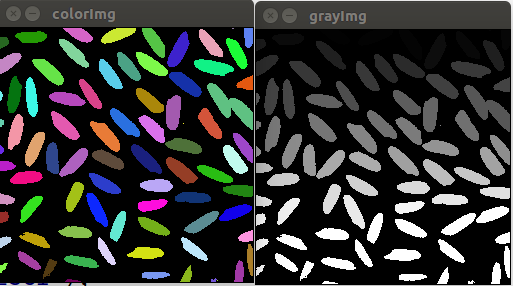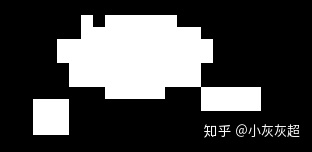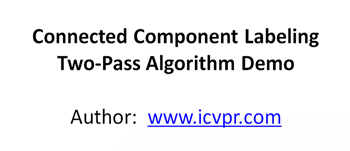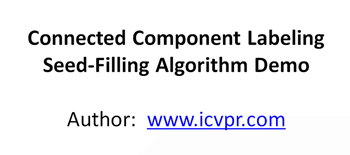• Matlab实现连通域标记算法求图像连通域连通域连通域标记算法 连通域 连通区域（Connected Component）一般是指图像中具有相同像素值且位置相邻的前景像素点组成的图像区域（Region，Blob）。连通区域分析（Connected...
Matlab实现连通域标记算法求图像连通域连通域连通域标记算法
连通域
连通区域（Connected Component）一般是指图像中具有相同像素值且位置相邻的前景像素点组成的图像区域（Region，Blob）。连通区域分析（Connected Component Analysis,Connected Component Labeling）是指将图像中的各个连通区域找出并标记。
连通区域分析是一种在CVPR和图像分析处理的众多应用领域中较为常用和基本的方法。例如：OCR识别中字符分割提取（车牌识别、文本识别、字幕识别等）、视觉跟踪中的运动前景目标分割与提取（行人入侵检测、遗留物体检测、基于视觉的车辆检测与跟踪等）、医学图像处理（感兴趣目标区域提取）、等等。也就是说，在需要将前景目标提取出来以便后续进行处理的应用场景中都能够用到连通区域分析方法，通常连通区域分析处理的对象是一张二值化后的图像。
连通域标记算法
选用图像：A=imread('d:\Temp\test.png');
[m,n]=size(A);
B=zeros(m,n);%标记矩阵
label=1; %不同连通域标记值
q=zeros(9999,2);%模拟队列
tail=1;
neighbour=[-1,-1;-1,0;-1,1;0,-1;0,1;1,-1;1,0;1,1];  %与某像素点相加得到该像素点的邻域像素点

for j=1:n
for i=1:m
if A(i,j)~=0&&B(i,j)==0
B(i,j)=label;
q(tail,:)=[i,j]; %用元组模拟队列，当前坐标入列
tail=tail+1;

for k=1:8
pix1=pix+neighbour(k,:);
if pix1(1)>=1&&pix1(1)<=m&&pix1(2)>=1&&pix1(2)<=n
if A(pix1(1),pix1(2))~=0&&B(pix1(1),pix1(2))==0
B(pix1(1),pix1(2))=label;
q(tail,:)=[pix1(1) pix1(2)];
tail=tail+1;
end
end
end
end
label=label+1;
tail=1;
end
end
end
imshow(mat2gray(B));


代码效果：展开全文matlab 图像识别 算法
• 图像处理的算法，可以用递归的方法标记连通域，能快速实现二值图像的连通域标记。
• 二值图像连通域二值图像分析最基础的也是最重要的方法之一就是连通域标记，它是所有二值图像分析的基础。它通过对二值图像中目标像素的标记，让每个单独的连通区域形成一个被标识的块，进一步的我们就可以获取这些块...
二值图像连通域二值图像分析最基础的也是最重要的方法之一就是连通域标记，它是所有二值图像分析的基础。它通过对二值图像中目标像素的标记，让每个单独的连通区域形成一个被标识的块，进一步的我们就可以获取这些块的轮廓、外接矩形、质心、不变矩等几何参数。 连通区域的定义一般有两种，分为4邻接和8邻接。下面这幅图中，如果考虑4邻接，则有3个连通域，8邻接则是2个连通域。从连通区域的定义可以知道，一个连通域是由具有相同像素值的相邻像素组成像素集合，因此，我们就可以通过这两个条件在图像中寻找连通区域，对于找到的每个连通域，我们赋予其一个唯一的标识( Label )，以区别其他连通域。连通域分析的基本算法有两种： 1) Two-Pass 两遍扫描 2) Seed-Filling 种子填充法。Two-Pass 算法两遍扫描法( Two-Pass )，正如其名，指的就是通过扫描两遍图像，将图像中存在的所有连通域找出并标记。(1)第一次扫描：访问当前像素 B(x,y) ，如果 B(x,y) == 1：a、如果 B(x,y) 的领域中标签值都为0，则赋予 B(x,y) 一个新的 label ：label += 1， B(x,y) = label；b、如果B(x,y)的领域中有像素值 > 1的像素Neighbors：1)将Neighbors中的最小值赋予给 B(x,y) :B(x,y) = min{Neighbors}2)记录Neighbors中各个值(label)之间的相等关系，即这些值(label)同属同一个连通区域；(2)第二次扫描：访问当前像素 B(x,y) ，如果 B(x,y) > 1：a、找到与 label = B(x,y) 同属相等关系的一个最小 label 值，赋予给 B(x,y) ；完成扫描后，图像中具有相同 label 值的像素就组成了同一个连通区域。另外，我在代码实现的过程中想到另外一种 Two-Pass 的方式(即扫描两遍图像的方式)实现，就是第二次扫描与 (1) 同样的过程,只是方向换成从右下到左上。我后面的 Two-Pass 代码是使用我自己想到的方法实现的，自己使用了几个例子测试了下，目前没出现啥问题。Seed-Filling 算法种子填充方法来源于计算机图形学，常用于对某个图形进行填充。它基于区域生长算法。我的理解就是递归遍历。附上两种方法的 Python 的实现import cv2import numpy as np# 4邻域的连通域和 8邻域的连通域# [row, col]NEIGHBOR_HOODS_4 = TrueOFFSETS_4 = [[0, -1], [-1, 0], [0, 0], [1, 0], [0, 1]]NEIGHBOR_HOODS_8 = FalseOFFSETS_8 = [[-1, -1], [0, -1], [1, -1],[-1,  0], [0,  0], [1,  0],[-1,  1], [0,  1], [1,  1]]def reorganize(binary_img: np.array):index_map = []points = []index = -1rows, cols = binary_img.shapefor row in range(rows):for col in range(cols):var = binary_img[row][col]if var < 0.5:continueif var in index_map:index = index_map.index(var)num = index + 1else:index = len(index_map)num = index + 1index_map.append(var)points.append([])binary_img[row][col] = numpoints[index].append([row, col])return binary_img, pointsdef neighbor_value(binary_img: np.array, offsets, reverse=False):rows, cols = binary_img.shapelabel_idx = 0rows_ = [0, rows, 1] if reverse == False else [rows-1, -1, -1]cols_ = [0, cols, 1] if reverse == False else [cols-1, -1, -1]for row in range(rows_, rows_, rows_):for col in range(cols_, cols_, cols_):label = 256if binary_img[row][col] < 0.5:continuefor offset in offsets:neighbor_row = min(max(0, row+offset), rows-1)neighbor_col = min(max(0, col+offset), cols-1)neighbor_val = binary_img[neighbor_row, neighbor_col]if neighbor_val < 0.5:continuelabel = neighbor_val if neighbor_val < label else labelif label == 255:label_idx += 1label = label_idxbinary_img[row][col] = labelreturn binary_img# binary_img: bg-0, object-255; intdef Two_Pass(binary_img: np.array, neighbor_hoods):if neighbor_hoods == NEIGHBOR_HOODS_4:offsets = OFFSETS_4elif neighbor_hoods == NEIGHBOR_HOODS_8:offsets = OFFSETS_8else:raise ValueErrorbinary_img = neighbor_value(binary_img, offsets, False)binary_img = neighbor_value(binary_img, offsets, True)return binary_imgdef recursive_seed(binary_img: np.array, seed_row, seed_col, offsets, num, max_num=100):rows, cols = binary_img.shapebinary_img[seed_row][seed_col] = numfor offset in offsets:neighbor_row = min(max(0, seed_row+offset), rows-1)neighbor_col = min(max(0, seed_col+offset), cols-1)var = binary_img[neighbor_row][neighbor_col]if var < max_num:continuebinary_img = recursive_seed(binary_img, neighbor_row, neighbor_col, offsets, num, max_num)return binary_img# max_num 表示连通域最多存在的个数def Seed_Filling(binary_img, neighbor_hoods, max_num=100):if neighbor_hoods == NEIGHBOR_HOODS_4:offsets = OFFSETS_4elif neighbor_hoods == NEIGHBOR_HOODS_8:offsets = OFFSETS_8else:raise ValueErrornum = 1rows, cols = binary_img.shapefor row in range(rows):for col in range(cols):var = binary_img[row][col]if var <= max_num:continuebinary_img = recursive_seed(binary_img, row, col, offsets, num, max_num=100)num += 1return binary_imgif __name__ == "__main__":binary_img = np.zeros((4, 7), dtype=np.int16)index = [[0, 2], [0, 5],[1, 0], [1, 1], [1, 2], [1, 4], [1, 5], [1, 6],[2, 2], [2, 5],[3, 1], [3, 2], [3, 4], [3, 6]]for i in index:binary_img[i, i] = np.int16(255)print("原始二值图像")print(binary_img)print("Two_Pass")binary_img = Two_Pass(binary_img, NEIGHBOR_HOODS_8)binary_img, points = reorganize(binary_img)print(binary_img, points)print("Seed_Filling")binary_img = Seed_Filling(binary_img, NEIGHBOR_HOODS_8)binary_img, points = reorganize(binary_img)print(binary_img, points)
展开全文• 连通域代码可以看看-二值图像连通域标记算法与代码_收藏.docx 给你们看看 赚积分matlab
• 二值图像连通域二值图像分析最基础的也是最重要的方法之一就是连通域标记，它是所有二值图像分析的基础。它通过对二值图像中目标像素的标记，让每个单独的连通区域形成一个被标识的块，进一步的我们就可以获取这些块...二值图像连通域二值图像分析最基础的也是最重要的方法之一就是连通域标记，它是所有二值图像分析的基础。它通过对二值图像中目标像素的标记，让每个单独的连通区域形成一个被标识的块，进一步的我们就可以获取这些块的轮廓、外接矩形、质心、不变矩等几何参数。 连通区域的定义一般有两种，分为4邻接和8邻接。下面这幅图中，如果考虑4邻接，则有3个连通域，8邻接则是2个连通域。从连通区域的定义可以知道，一个连通域是由具有相同像素值的相邻像素组成像素集合，因此，我们就可以通过这两个条件在图像中寻找连通区域，对于找到的每个连通域，我们赋予其一个唯一的标识（ Label ），以区别其他连通域。连通域分析的基本算法有两种： 1) Two-Pass 两遍扫描 2) Seed-Filling 种子填充法。  Two-Pass 算法两遍扫描法（ Two-Pass ），正如其名，指的就是通过扫描两遍图像，将图像中存在的所有连通域找出并标记。（1）第一次扫描：
访问当前像素 B(x,y) ，如果 B(x,y) == 1：
a、如果 B(x,y) 的领域中标签值都为0，则赋予 B(x,y) 一个新的 label ：
label += 1， B(x,y) = label；
b、如果B(x,y)的领域中有像素值 > 1的像素Neighbors：
1）将Neighbors中的最小值赋予给 B(x,y) :
B(x,y) = min{Neighbors}
2）记录Neighbors中各个值（label）之间的相等关系，即这些值（label）同属同一个连通区域；

（2）第二次扫描：
访问当前像素 B(x,y) ，如果 B(x,y) > 1：
a、找到与 label = B(x,y) 同属相等关系的一个最小 label 值，赋予给 B(x,y) ；
完成扫描后，图像中具有相同 label 值的像素就组成了同一个连通区域。

另外，我在代码实现的过程中想到另外一种 Two-Pass 的方式(即扫描两遍图像的方式)实现，就是第二次扫描与 (1) 同样的过程,只是方向换成从右下到左上。我后面的 Two-Pass 代码是使用我自己想到的方法实现的，自己使用了几个例子测试了下，目前没出现啥问题。Seed-Filling 算法种子填充方法来源于计算机图形学，常用于对某个图形进行填充。它基于区域生长算法。我的理解就是递归遍历。附上两种方法的 Python 的实现import cv2
import numpy as np

# 4邻域的连通域和 8邻域的连通域
# [row, col]
NEIGHBOR_HOODS_4 = True
OFFSETS_4 = [[0, -1], [-1, 0], [0, 0], [1, 0], [0, 1]]

NEIGHBOR_HOODS_8 = False
OFFSETS_8 = [[-1, -1], [0, -1], [1, -1],
[-1,  0], [0,  0], [1,  0],
[-1,  1], [0,  1], [1,  1]]

def reorganize(binary_img: np.array):
index_map = []
points = []
index = -1
rows, cols = binary_img.shape
for row in range(rows):
for col in range(cols):
var = binary_img[row][col]
if var < 0.5:
continue
if var in index_map:
index = index_map.index(var)
num = index + 1
else:
index = len(index_map)
num = index + 1
index_map.append(var)
points.append([])
binary_img[row][col] = num
points[index].append([row, col])
return binary_img, points

def neighbor_value(binary_img: np.array, offsets, reverse=False):
rows, cols = binary_img.shape
label_idx = 0
rows_ = [0, rows, 1] if reverse == False else [rows-1, -1, -1]
cols_ = [0, cols, 1] if reverse == False else [cols-1, -1, -1]
for row in range(rows_, rows_, rows_):
for col in range(cols_, cols_, cols_):
label = 256
if binary_img[row][col] < 0.5:
continue
for offset in offsets:
neighbor_row = min(max(0, row+offset), rows-1)
neighbor_col = min(max(0, col+offset), cols-1)
neighbor_val = binary_img[neighbor_row, neighbor_col]
if neighbor_val < 0.5:
continue
label = neighbor_val if neighbor_val < label else label
if label == 255:
label_idx += 1
label = label_idx
binary_img[row][col] = label
return binary_img

# binary_img: bg-0, object-255; int
def Two_Pass(binary_img: np.array, neighbor_hoods):
if neighbor_hoods == NEIGHBOR_HOODS_4:
offsets = OFFSETS_4
elif neighbor_hoods == NEIGHBOR_HOODS_8:
offsets = OFFSETS_8
else:
raise ValueError

binary_img = neighbor_value(binary_img, offsets, False)
binary_img = neighbor_value(binary_img, offsets, True)

return binary_img

def recursive_seed(binary_img: np.array, seed_row, seed_col, offsets, num, max_num=100):
rows, cols = binary_img.shape
binary_img[seed_row][seed_col] = num
for offset in offsets:
neighbor_row = min(max(0, seed_row+offset), rows-1)
neighbor_col = min(max(0, seed_col+offset), cols-1)
var = binary_img[neighbor_row][neighbor_col]
if var < max_num:
continue
binary_img = recursive_seed(binary_img, neighbor_row, neighbor_col, offsets, num, max_num)
return binary_img

# max_num 表示连通域最多存在的个数
def Seed_Filling(binary_img, neighbor_hoods, max_num=100):
if neighbor_hoods == NEIGHBOR_HOODS_4:
offsets = OFFSETS_4
elif neighbor_hoods == NEIGHBOR_HOODS_8:
offsets = OFFSETS_8
else:
raise ValueError

num = 1
rows, cols = binary_img.shape
for row in range(rows):
for col in range(cols):
var = binary_img[row][col]
if var <= max_num:
continue
binary_img = recursive_seed(binary_img, row, col, offsets, num, max_num=100)
num += 1
return binary_img

if __name__ == "__main__":
binary_img = np.zeros((4, 7), dtype=np.int16)
index = [[0, 2], [0, 5],
[1, 0], [1, 1], [1, 2], [1, 4], [1, 5], [1, 6],
[2, 2], [2, 5],
[3, 1], [3, 2], [3, 4], [3, 6]]
for i in index:
binary_img[i, i] = np.int16(255)

print("原始二值图像")
print(binary_img)

print("Two_Pass")
binary_img = Two_Pass(binary_img, NEIGHBOR_HOODS_8)
binary_img, points = reorganize(binary_img)
print(binary_img, points)

print("Seed_Filling")
binary_img = Seed_Filling(binary_img, NEIGHBOR_HOODS_8)
binary_img, points = reorganize(binary_img)
print(binary_img, points)
展开全文• opencv连通域学习连通域 连通域 图像的连通域是指图像中具有相同像素值并且位置相邻的像素组成的区域，连通域分析是指在图像中寻找彼此相互独立的连通域并将其标记出来。提取图像中不同的连通域是图像中较为常用的...
opencv连通域学习连通域
连通域
图像的连通域是指图像中具有相同像素值并且位置相邻的像素组成的区域，连通域分析是指在图像中寻找彼此相互独立的连通域并将其标记出来。提取图像中不同的连通域是图像中较为常用的方法。例如在车牌识别、文字识别、目标检测等领域对感兴趣区域分割与识别。一般情况下，一个连通域内只包含一个像素值，因此为了防止像素值波动对提取不同连通域的影响，连通域分析常处理的是二值化后的图像。
图像中两个像素相邻有两种定义方式，分别是4-领域和8-领域。根据两个像素相邻的定义方式不同，得到的连通域也不相同，因此在分析连通域的同时，一定要声明是在哪种领域条件下分析得到的结果。常用的图像领域分析法有两遍扫描法和种子填充法。两遍扫描法会遍历两次图像，第一次遍历图像时会给每个非0像素赋予一个数字标签，当某个像素的上方和左侧领域内的像素已经有数字标签时，取两者中的最小值作为当前像素的标签，否则赋予当前像素一个新的数字标签。第一次遍历图像的时候同一个连通域可能会被赋予一个或者多个不同的标签，如下图所示，因此第二次遍历需要将这些属于同一个连通域的不同标签合并，最后实现同一个领域内的所有像素具有相同的标签。种子填充法源于计算机图像学，常用于对某些图形进行填充。该方法首先将所有非0像素放到一个集合中，之后在集合中随机选出一个像素作为种子像素，根据领域关系不断扩充种子像素所在的连通域，并在集合中删除掉扩充出的像素，直到种子像素所在的连通域无法扩充，之后再从集合中随机选取一个像素作为新的种子像素，重复上述过程直到集合中没有像素。
opencv4提供了用于提取图像中不同连通域的connectedComponents()函数，该函数用于计算二值图像中连通域的个数，并在图像中将不同的连通域用不同的数字标签标记出来，其中标签0表示图像中的背景区域，同时函数具有一个int类型的返回数据，用于表示图像中连通域的数目。函数的第一个参数时待标记连通域的输入图像，函数要求输入图像必须是数据类型为CV_8U的单通道灰度图像，而且最好是经过二值化的二值图像。函数第二个参数是标记连通域后的输出图像，图像尺寸与第一个参数的输入图像尺寸相同，图像的数据类型与函数的第四个参数相关。函数第三个参数是统计连通域时选择的领域种类，函数支持两种领域，分别用4表示4-领域，8表示8-领域。函数第四个参数为输出图像的数据类型，可以选择的参数为CV_32和CV_16U两种。函数的最后一个参数时标记连通域时使用算法的标志，目前只支持Grana(BBDT)和Wu(SAUF)两种算法。
上述函数原型的所有参数都没有默认值，在调用时需要设置全部参数，增加了使用的复杂程度，因此opencv4提供了connectedComponents()函数的简易原型，减少了参数数量以及部分参数增加了默认。


展开全文机器学习
• 通过以上函数，确定出二值图片中的一些连通域，接下来通过一些筛选条件，即连通域宽高比的无用连通域过滤掉，想把无用的连通域设置为背景色（黑色）。请问如何将这些区域设置为黑色？？？？？？ 已有思路：得到的...人工智能 自然语言处理
• 对一幅二值图像里面的部分连通域进行膨胀操作，要求膨胀后不能与其他连通域合并成一个，应该怎么判断膨胀后是否粘连呢 C币用完了发不了悬赏了 抱歉开发语言
• 图像连通域连通域图像的连通域是指图像中具有相同像素值并且位置相邻的像素组成的区域，连通域分析是指在图像中寻找出彼此互相独立的连通域并将其标记出来。提取图像中不同的连通域是图像处理中较为常用的方法，例如...
• 基于快速连通域标记的车牌字符分割王冠;敖志刚;刘永跃;王真军【期刊名称】《计算机与现代化》【年(卷),期】2007(000)006【摘要】对车牌图像的二值图进行连通域提取可以解决倾斜带来的问题.提出了一种基于快速连通域...
• https://github.com/becomequantum/kryon还做了个算法演示动画：https://www.bilibili.com/video/av26067000《FPGA图像处理基本技巧》：https://zhuanlan.zhihu.com/p/38946857《FPGA实现的实时流水线连通域标记算法...
• 之前写过连通域的分割算法，这次就用该算法来分割字符。假设有如下图片，首先基于连通域进行分割：import numpy as npimport cv2from matplotlib import pyplot as pltclass ImgSplit(): def __init__(self,path): ...
• 基于连通域快速文字图像分割算法基于连通域快速文字图像分割算法摘 要：针对文本图像中的文字难以提取分割的问题，文章提出了一种基于连通域的算法。算法通过连通域阀值分析，将文本块联通，继而将文字成功分割。...
• 基于连通域的版面分割研究郭丽;黄元元;杨静宇【摘要】版面分割是OCR(光学字符识别)系统的重要组成部分,在将印刷文档转换为电子文档的过程中是必不可少的.该文对基于连通域的版面分割方法进行研究,首先用动态聚类法...
• L = bwlabel(BW,n)返回一个和BW大小相同的L矩阵，包含了标记了BW中每个连通区域的类别标签，这些标签的值为1、2、num(连通区域的个数)。...一些连通域处理函数，转自AmazingJack的专栏：http://m.blog.csdn...
• 做材料缺陷分析的时候，会用刀三维连通域分析这个算法，作为一个不入流的JS码农，算法我是万万不会自己去写的，而且还是用python去写。不过好在，确实有人写出了很成功的库，可以得以引用，我这里就来重点介绍一个这...
• 机器学习AI算法工程 公众号：datayx二值图像连通域二值图像分析最基础的也是最重要的方法之一就是连通域标记，它是所有二值图像分析的基础。它通过对二值图像中目标像素的标记，让每个单独的连通区域形成一个被标识...
• 1.思路是用深度遍历，对图片进行二值化处理，先找到一个黑色像素，然后对这个像素的周围8个像素进行判断，如果没有访问过，就保存...def cfs(img):"""传入二值化后的图片进行连通域分割"""pixdata = img.load()w,h =...
• 4连通域边界填充算法和8连通域边界填充算法 //边界填充算法 //边界填充算法-4连通域 void boundaryFill4(int x,int y,int fillColor,int borderColor) { int interiorColor; getPixel(x,y,interiorColor); if...
• 根据连通域的形状和进邻连接等特征筛选，合并连通域。对于文档而言，连文献：《复杂车辆图像中的车牌定位与字符分割方法》:1.先搜索标记二值图像的所有连通域2.根据连通域的形状和进邻连接等特征筛选，合并连通域。...
• 连通域处理函数的原型前言在图像处理过程中，经常会遇到这样一部分图像，图像的整体部分如果人来看的话一眼就能看出，但是它的内部由于有各种小缺口，导致断开了，这样在 计算机“眼”里就被认为是断开的，为了使...
• 基于改进连通域算法的车牌字符分割方法朱亚萍，邱锦山，杨成忠【摘要】摘要：为了解决车牌字符分割中不连通汉字分割、粘连字符分割以及竖直边框干扰等问题，提出了一种基于改进连通域算法的车牌字符分割方法....
• 连通域分析其实是一个路径搜索问题，搜索方式就看联通的规则(4联通：上下左右算是联通，8联通：上下左右还有四个对角)01矩阵背景是0，黑色的，有色区域是1，白色的，从图像的左上角(最外围的边要去掉)进行遍历，将...
• ## 连通域求解

千次阅读 2018-03-18 17:25:56
图像处理中连通域指由前景相同像素，并且相同像素邻接的像素组成的域。图像处理中一般都是对二值图像（1白色，0为黑色，一般前景为0黑色）做连通域分析。连通域分析指把连通域找出来并且标记出来。连通域标记方法：...图像
• python中验证码连通域分割的方法详解这篇文章主要给大家介绍了关于python中验证码连通域分割的相关资料，文中通过示例代码介绍的非常详细，对大家学习或者使用python具有一定的参考学习价值，需要的朋友们下面随着小...
• 作者：徐港，赵恬悦，蒋赏，高德军摘要：针对传统裂缝图像信息提取方法的局限性，提出了一种基于多种连通域特征的工程结构表面裂缝提取方法．在采用最大类间方差(Otsu)法对原始图像进行初始分割的基础上，对裂缝图像......